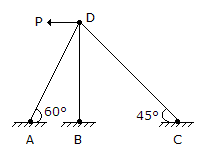# Civil Engineering - Theory of Structures - Discussion

Discussion Forum : Theory of Structures - Section 4 (Q.No. 16)
16.
The degree of indeterminacy of the frame in the given figure, iszero
1
2
3
Explanation:
No answer description is available. Let's discuss.
Discussion:
15 comments Page 1 of 2.

Sowmya said:   9 years ago
m+r-2j = 3+6-8 = 1.

EMAD ERNEST said:   8 years ago
We have x & y reaction at each of a, b & c giving 6 unknowns. Then the correct answer must be 3. But if the question was "Number of side ways" then the answer is 1.

Akash said:   7 years ago
M + r - 2j, for true.

Mamun said:   5 years ago
Here m+r-2j = 3+6-8 = 1.

Aman gupta said:   5 years ago
Its frame not a trus so formula= 3m+re-3j.

(3*3) +4- (3*4) =1.

Getasew said:   5 years ago
m = 3.
r = 6,
j = 4,
= 6+3-2*4.
= 1.

Abhik said:   5 years ago
M+r -2j is applied to truss.
(1)

Saqib said:   4 years ago
2j-r = 2x4-9 = 1.

Saqib said:   4 years ago
M+r-2j= 3+6-2x4 = 1.

Waleed said:   3 years ago
It should be zero.